Refer to our Texas Go Math Grade 4 Answer Key Pdf to score good marks in the exams. Test yourself by practicing the problems from Texas Go Math Grade 4 Unit 5 Assessment Answer Key.

Vocabulary

frequency table
frequency
dot plot
stem-and-leaf plot

Choose the best term from the box.

Question 1.
A ________________ shows groups of data arranged by place value.

Question 2.
A ________________ is a graph that shows the frequency of data along a number line.

Question 3.
The ________________ is the number of times data occurs.

Concepts and Skills

Use the Number of Apples Picked table for 4-6.

Janet and her classmates went to an apple orchard to pick apples. Janet recorded the number of apples some of her classmates picked.Question 4.
If Janet represents this data in a stem-and-leaf plot, how many leaves would there be? _______________
There are 15 leaves if he represents this data in a stem-and-leaf plot. This will be explained below:
The stem-and-leaf plot:
0| 7  9
1| 2  2  8   8   8
2| 0  0  0   0   4   5
3| 5  5
Count all the leaves.
The total leaves are 15.

Question 5.
Janet wants to make a frequency table from this data.
Which number of apples picked has the greatest frequency? _______________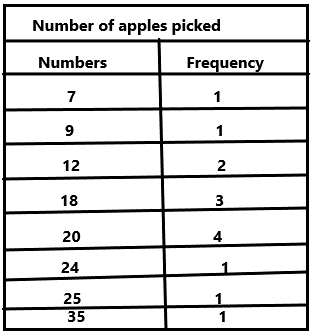The highest frequency is 4 and the number is 20.

Question 6.
Janet decided that instead of a frequency table, she wants to make a dot plot. how many dots will he above the number 20? _______________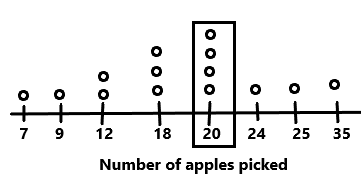The dots above the 20 is 4.

Use the Amount of Raisins Used table for 7-8.

Barry and his classmates made trail mix. They each used different amounts of raisins. Barry recorded the amounts of raisins that the classmates used.Question 7.
Barry wants to make a dot plot with the data.
How many total dots will go above $$\frac{1}{4}$$ and $$\frac{3}{4}$$? ___________
Explanation: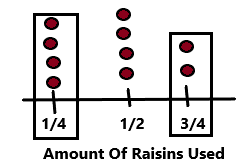The dots above $$\frac{1}{4}$$ =4
The dots above $$\frac{3}{4}$$=2
total dots will go above $$\frac{1}{4}$$ and $$\frac{3}{4}$$=4+2=6.

Question 8.
If Barry created a frequency table with this data,
what number would be in the frequency column for $$\frac{1}{2}$$? ___________
Explanation: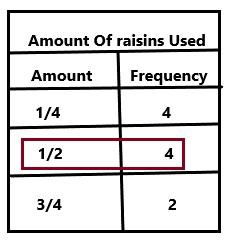The number would be in the frequency column for $$\frac{1}{2}$$ is 4. I circled in the above diagram.

Use the Roller coasters Ridden dot plot for 9-10.

The campers from a summer camp went on a field trip to an amusement park. During the trip, Vicki recorded how many roller coasters each camper went on. She recorded the data in a dot plot.Question 9.
How many campers rode either 3 or 5 roller coasters?
(A) 7
(B) 8
(C) 4
(D) 11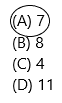Explanation: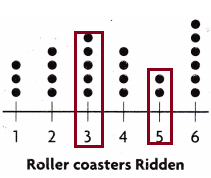The number of dots above 3 is 5
The number of dots above 5 is 2
The total number of dots on both rollers coasters ridden is 5+2=7.

Question 10.
How many campers rode 2 or more roller coasters?
(A) 18
(B) 25
(C) 19
(D) 21Explanation:Add all the dots above 2 , 3, 4, 5, 6: 4+5+4+2+6=21.

Use the Length of Leaves frequency table for 11-12.

Several scientists went to a field to collect various leaves. When they got back to the lab, they measured each leaf, in feet. They recorded the data in a frequency table.Question 11.
How many leaves were less than $$\frac{3}{8}$$ft in length?
(A) 19
(B) 30
(C) 7
(D) 9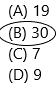Explanation:The length of leaves is 1/8 and its frequency is 11.
The length of leaves is 1/4 and its frequency is 19.
The question asked was the number of leaves was less than $$\frac{3}{8}$$ft in length. To get the answer to add the length of leaves 1/8 and 1/4 and their frequencies:11+9=30.
Because 1/8 and 1/4 are less than 3/8.

Question 12.
How many more leaves are $$\frac{1}{4}$$ft than $$\frac{3}{8}$$ft and $$\frac{1}{2}$$ft combined?
(A) 19
(B) 30
(C) 7
(D) 9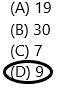Explanation:
The leaves of $$\frac{1}{4}$$ft and its frequency=19
The leaves of $$\frac{3}{8}$$ft and $$\frac{1}{2}$$ft combined and its frequencies are: 7+3=10.
We need to find out the number of more leaves present in 1/4 than 3/8 and 1/2=19-10=9.

Question 13.
Sasha asked her friends how many books they read during their two week vacation. She recorded their responses in a frequency table. How many of her friends read 1 or more books during their vacation?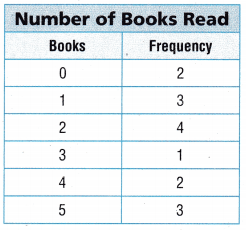(A) 8
(B) 15
(C) 13
(D) 12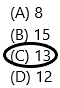Explanation:
Add all the frequencies present from 1 and above.
The number of her friends who read 1 or more books during their vacation:
3+4+1+2+3=13.Use the Weight of Rocks dot plot for 14-15.

Frank wants to record the weights of the different rocks in his collection. He weighs each rock in pounds and records the data in a dot plot.Question 14.
How many rocks does Frank have that weigh more than a pound but less than 1.7 pounds?
(A) 9
(B) 7
(C) 14
(D) 10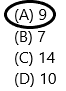Explanation:
More than a pound means we need to count from 1.4 and less than 1.7 means we need to count up to 1.6.
Total rocks=3+2+4=9 (1.4, 1.5, 1.6).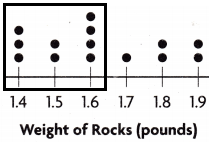Question 15.
How many rocks does Frank have in his collection?
(A) 1o
(B) 11
(C) 13
(D) 14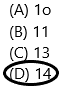Explanation:
Add all the dot plots from 1.4 to 1.9.
The dot plots are 1.4, 1.5, 1.6, 1.7, 1.8, 1.9 and their dots are 3+2+4+1+2+2=14.

Question 16.
Kerry kept track of the time he spent on the computer in a frequency table. How many more times did Kerry spend 4 hours or less than he spent 5 hours or more using the computer?(A) 17
(B) 20
(C) 19
(D) 23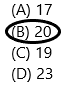Explanation:
The Kerry spend 4 hours or less=13+10+12=35
The Kerry spend 5 hours or more=5+9+1=15
The number of more times he spends 4 hours or less than 5 hours or more=35=15=20

Question 17.
Jackson asked the 3rd grade students to find the distance that they live from the school, in miles. Jackson recorded the students answers in a frequency table. How many total students live less than mile or more than 2 miles from the school?(A) 18
(B) 20
(C) 26
(D) Not hereExplanation:
The number of students who live less than a mile to school that is 0.4 and 0.8=8+4=12
The number of students who live more than 2 miles to school that is 2.1, 2.2, 2.4 =2+1+4=7
Total:12+7=19. But there is no option on 19.

Use the Number of Goals Scored stem-and-leaf plot for 18-19.

During the soccer season, Dylan kept record of the number of goals scored by each team. At the end of the season, Dylan made a stern-and-leaf plot to show the total number of goals scored by each team.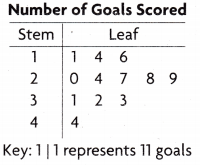Question 18.
How many teams scored less than 20 goals during the season?
(A) 4
(B) 3
(C) 8
(D) 5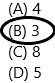Explanation:
we need to count the leaves which are having less than 20. This representation will be shown in the diagram.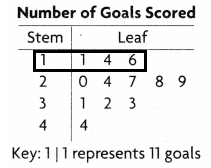1| 1 represents 11 goals
1| 4 represents 14 goals
1| 6 represents 16 goals.
These are less than 20 goals.

Question 19.
How many more teams scored less than 30 goals than scored more than 30 goals during the season?
(A) 3
(B) 8
(C) 5
(D) 4Explanation: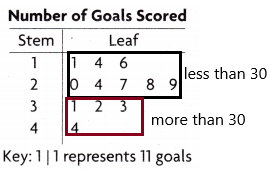The number of teams scored less than 30 are 8.
The number of teams scored more than 30 are 4
The teams scored less than 30 goals than scored more than 30 goals during the season:8-4=4.

Use the Time Spent Practicing dot plot for 20-21.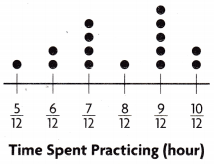Question 20.
Chad recorded the number of times he spent practicing his saxophone. How many more times did Chad practice for $$\frac{9}{12}$$ hour or more than he practiced for $$\frac{6}{12}$$ hour or less?
(A) 2
(B) 4
(C) 3
(D) 6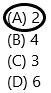Explanation:
The dots having on 9/12 are 5
The dots having on 6/12 and 5/12 are 2+1=3
The number of more times he practised for 9/12 than 6/12 and less=5-3=2.

Question 21.
How many times did Chad practice for $$\frac{7}{12}$$ hour?
(A) 2
(B) 3
(C) 4
(D) 5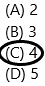Explanation:Count all the dots present above the 7/12. There are 4 dots present.

Question 22.
Brandon borrowed a book from the library. The data show the lengths of time Brandon read the book each day until he finished it. Explain how you would use the data to label and plot the dots on a dot plot. What is the difference between the longest time and shortest time Brandon spent reading the book?1. The representation must be lowest to highest.
2. Count and write the number of dots above the respective places.
3. The diagram representation is shown below:The dot plot of 1/4 is having 4 dots.
The dot plot of 1/2 is having 2 dots.
The dot plot of 3/4 is having 1 dot.
The dot plot of 1 is having 1 dot.
In the question asked was the difference between the longest time and shortest time Brandon spent reading the book.
The longest time is 1 hour the dot is 1.
The shortest time is 1/4 hour that’s the dot is 4.
The difference is 4-1=3 hours.

Scroll to Top